Publicité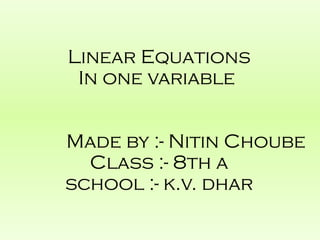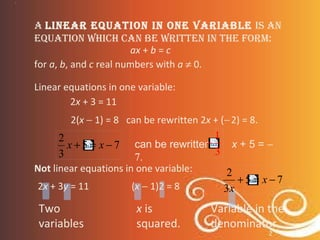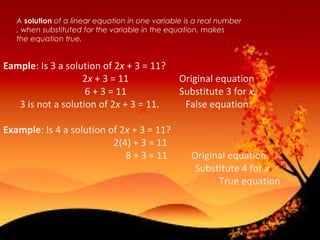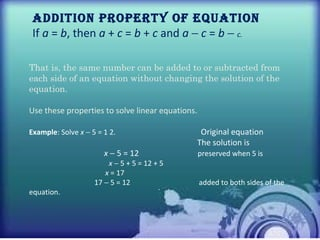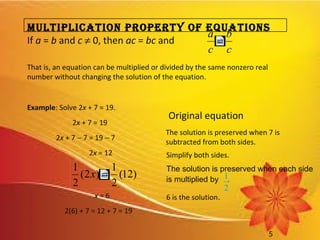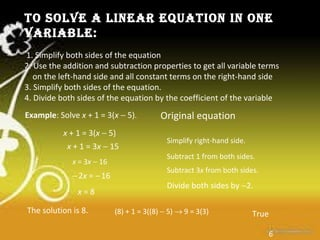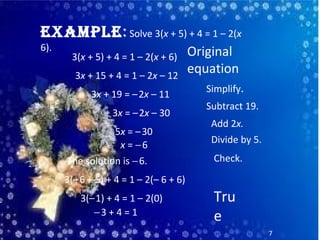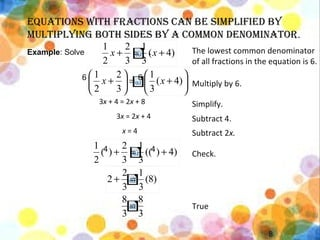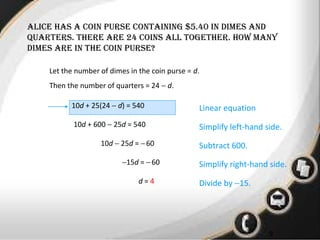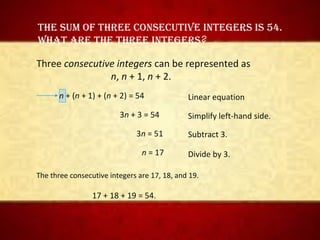1 sur 11
Publicité

### Linear Equation in one variable - Class 8 th Maths

1. Linear Equations In one variable Made by :- Nitin Choube Class :- 8th a school :- k.v. dhar
2. • 1 2 A lineAr equAtion in one vAriAble is An equAtion which cAn be written in the form: ax + b = c for a, b, and c real numbers with a ≠ 0. Linear equations in one variable: 2x + 3 = 11 2(x − 1) = 8 Not linear equations in one variable: 2x + 3y = 11 Two variables can be rewritten 2x + (−2) = 8. x is squared. Variable in the denominator (x − 1)2 = 8 can be rewritten x + 5 = − 7. 75 3 2 −=+ xx 3 1 − 75 3 2 −=+ x x
3. A solution of a linear equation in one variable is a real number , when substituted for the variable in the equation, makes the equation true.• 1 Eample: Is 3 a solution of 2x + 3 = 11? 2x + 3 = 11 Original equation 6 + 3 = 11 Substitute 3 for x. 3 is not a solution of 2x + 3 = 11. False equation Example: Is 4 a solution of 2x + 3 = 11? 2(4) + 3 = 11 8 + 3 = 11 Original equation Substitute 4 for x True equation
4. • 1 4 Addition ProPerty of equAtion If a = b, then a + c = b + c and a − c = b − c. . That is, the same number can be added to or subtracted from each side of an equation without changing the solution of the equation. Use these properties to solve linear equations. Example: Solve x − 5 = 1 2. Original equation The solution is x − 5 = 12 preserved when 5 is x − 5 + 5 = 12 + 5 x = 17 17 − 5 = 12 added to both sides of the equation.
5. • 1 5 MultiPlicAtion ProPerty of equAtions If a = b and c ≠ 0, then ac = bc and That is, an equation can be multiplied or divided by the same nonzero real number without changing the solution of the equation. Example: Solve 2x + 7 = 19. 2x + 7 = 19 2x + 7 − 7 = 19 − 7 2x = 12 x = 6 2(6) + 7 = 12 + 7 = 19 Original equation The solution is preserved when 7 is subtracted from both sides. Simplify both sides. 6 is the solution. The solution is preserved when each side is multiplied by . 2 1 c b c a = )12( 2 1 )2( 2 1 =x
6. • 1 6 Example: Solve x + 1 = 3(x − 5). x + 1 = 3(x − 5) x + 1 = 3x − 15 x = 3x − 16 −2x = −16 x = 8 The solution is 8. Original equation Simplify right-hand side. Subtract 1 from both sides. Subtract 3x from both sides. Divide both sides by −2. True(8) + 1 = 3((8) − 5) → 9 = 3(3) to solve A lineAr equAtion in one vAriAble: 1. Simplify both sides of the equation 2. Use the addition and subtraction properties to get all variable terms on the left-hand side and all constant terms on the right-hand side 3. Simplify both sides of the equation. 4. Divide both sides of the equation by the coefficient of the variable
7. • 1 7 ExamplE: Solve 3(x + 5) + 4 = 1 – 2(x 6). 3(x + 5) + 4 = 1 – 2(x + 6) 3x + 15 + 4 = 1 – 2x – 12 3x + 19 = –2x – 11 3x = –2x – 30 5x = –30 x = −6 The solution is −6. Original equation Simplify. Subtract 19. Add 2x. Divide by 5. Check. Tru e−3 + 4 = 1 3(–1) + 4 = 1 – 2(0) 3(–6 + 5) + 4 = 1 – 2(– 6 + 6)
8. • 1 8 Equations with fractions can bE simplifiEd by multiplying both sidEs by a common dEnominator. The lowest common denominator of all fractions in the equation is 6. 3x + 4 = 2x + 8 3x = 2x + 4 x = 4 Multiply by 6. Simplify. Subtract 4. Subtract 2x. Check. True Example: Solve . 4 4 6 6 )4( 3 1 3 2 2 1 +=+ xx 4))(( 3 1 3 2 )( 2 1 +=+ )8( 3 1 3 2 2 =+ 3 8 3 8 =       +=      + )4( 3 1 3 2 2 1 xx
9. • 1 9 alicE has a coin pursE containing \$5.40 in dimEs and quartErs. thErE arE 24 coins all togEthEr. how many dimEs arE in thE coin pursE? Let the number of dimes in the coin purse = d. Then the number of quarters = 24 − d. 10d + 25(24 − d) = 540 Linear equation 10d + 600 − 25d = 540 10d − 25d = −60 −15d = −60 d = 4 Simplify left-hand side. Subtract 600. Simplify right-hand side. Divide by −15.
10. • 1 The sum of Three consecuTive inTegers is 54. WhaT are The Three inTegers? Three consecutive integers can be represented as n, n + 1, n + 2. n + (n + 1) + (n + 2) = 54 3n + 3 = 54 3n = 51 n = 17 Simplify left-hand side. Subtract 3. Divide by 3. The three consecutive integers are 17, 18, and 19. 17 + 18 + 19 = 54. Linear equation
11. thank you
Publicité